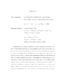## An inexact interior-point algorithm for conic convex optimization problems

 dc.contributor.advisor Tits, Andre L en_US dc.contributor.advisor O'Leary, Dianne P en_US dc.contributor.author Schurr, Simon Peter en_US dc.contributor.department Applied Mathematics and Scientific Computation en_US dc.contributor.publisher Digital Repository at the University of Maryland en_US dc.contributor.publisher University of Maryland (College Park, Md.) en_US dc.date.accessioned 2006-11-01T06:32:23Z dc.date.available 2006-11-01T06:32:23Z dc.date.issued 2006-10-02 en_US dc.description.abstract In this dissertation we study an algorithm for convex optimization problems in conic form. (Without loss of generality, any convex problem can be written in conic form.) Our algorithm belongs to the class of interior-point methods (IPMs), which have been associated with many recent theoretical and algorithmic advances in mathematical optimization. In an IPM one solves a family of slowly-varying optimization problems that converge in some sense to the original optimization problem. Each problem in the family depends on a so-called barrier function that is associated with the problem data. Typically IPMs require evaluation of the gradient and Hessian of a suitable (self-concordant'') barrier function. In some cases such evaluation is expensive; in other cases formulas in closed form for a suitable barrier function and its derivatives are unknown. We show that even if the gradient and Hessian of a suitable barrier function are computed inexactly, the resulting IPM can possess the desirable properties of polynomial iteration complexity and global convergence to the optimal solution set. In practice the best IPMs are primal-dual methods, in which a convex problem is solved together with its dual, which is another convex problem. One downside of existing primal-dual methods is their need for evaluation of a suitable barrier function, or its derivatives, for the dual problem. Such evaluation can be even more difficult than that required for the barrier function associated with the original problem. Our primal-dual IPM does not suffer from this drawback---it does not require exact evaluation, or even estimates, of a suitable barrier function for the dual problem. Given any convex optimization problem, Nesterov and Nemirovski showed that there exists a suitable barrier function, which they called the universal barrier function. Since this function and its derivatives may not be available in closed form, we explain how a Monte Carlo method can be used to estimate the derivatives. We make probabilistic statements regarding the errors in these estimates, and give an upper bound on the minimum Monte Carlo sample size required to ensure that with high probability, our primal-dual IPM possesses polynomial iteration complexity and global convergence. en_US dc.format.extent 1246235 bytes dc.format.mimetype application/pdf dc.identifier.uri http://hdl.handle.net/1903/3977 dc.language.iso en_US dc.subject.pqcontrolled Mathematics en_US dc.subject.pquncontrolled conic convex optimization en_US dc.subject.pquncontrolled self-concordant barrier function en_US dc.subject.pquncontrolled interior-point method en_US dc.subject.pquncontrolled inexact algorithm en_US dc.subject.pquncontrolled polynomial time complexity en_US dc.subject.pquncontrolled universal barrier function en_US dc.title An inexact interior-point algorithm for conic convex optimization problems en_US dc.type Dissertation en_US
##### Original bundle
Now showing 1 - 1 of 1